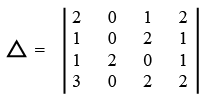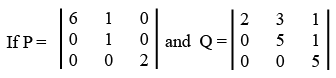# ISRO Scientist or Engineer Civil 2018

Instructions

For the following questions answer them individually

Question 71

# The value ofisQuestion 72

# A rectangular sheet of metal of length 6m and width 2 m is given. Four equal squares are removed from the corners. The sides of this sheet are now turned up to form an open rectangular box. The height of box such that the volume of the box is maximum will beQuestion 73

# The complete solution of the linear differential equation $$\frac{\text{d}^2z}{\text{d}t^2} + (p + q) \frac{\text{d}z}{\text{d}t} + pqz = 0$$Question 74

# The sequence $$\lim_{a \rightarrow \infty} \left[ 3 + (-1)^n\right]$$ isQuestion 75

#Then the product of determinant P and Q ( PQ ) has the valueQuestion 76

# The differential equation $$y = x \frac{\text{d}y}{\text{d}x} + \frac{x}{\frac{dy}{dx}}$$ isQuestion 77

# In a square matrix $$A = [ a_ij ],$$ if $$a_ij = − a_ji$$ for all i and j so that all the leading diagonal elements are zero, then the matrix is calledQuestion 78

# The difference of two complex numbers $$(x_1 + iy_1)$$ and $$(x_2 + iy_2)$$ isQuestion 79

# The value of $$\lim_{x \rightarrow 0} \frac {\log x}{\cot x}$$ isQuestion 80

# A box contains 5 red and 10 green balls. Eight (8) of them are placed in another box. The chances that the latter box contains 2 red and 6 green balls areOR